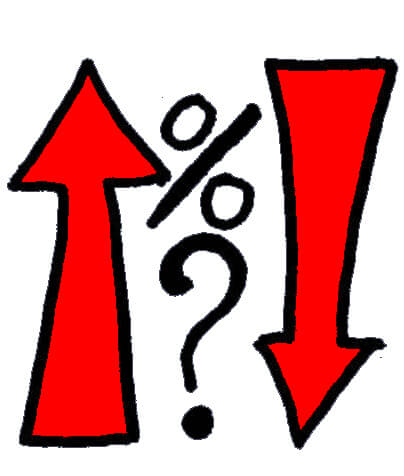# INTEREST-RATE RULES: Rules that Involve a Lagged Inter est-RateWe achieve improvements in household welfare if we generalize the family of simple Taylor rules to allow the funds rate to respond also to lagged values of itself. We thus consider generalized Taylor rules of the formwhere we now allow с to be greater than zero. This allows for interest rate smoothing, so that sustained changes in output and inflation lead to only gradual changes in interest rates. Actual policy in the United States and elsewhere seems to involve some degree of interest rate smoothing, though academic commentators have often questioned why this should be so. read more

Nor is there any reason to restrict attention to the case 0 < с < 1, though it is only in that case that the policy rule can be described as involving partial adjustment toward a “target” funds rate that depends upon current output and inflation, as assumed for example in Clarida et al (1997). An alternative is to follow Fuhrer and Moore (1995) and model U.S. interest rates by supposing that с is equal to one, so that it is changes in the funds rate, rather than the level of the funds rate, that respond to deviations in inflation and output from their typical levels. Policy proposals of this kind are considered elsewhere in this volume with rules Aq and Bq in Table 1 being examples of such rules. We find that policies that involve values of с even greater than one often result in determinate rational expectations equilibria in our model, and so we consider arbitrary positive values of c. In fact, rules with с > 1 turn out to possess an important advantage, and this is one of our most important findings.To gain some insight into the consequences of varying c, we set b equal to zero and discuss contour plots in the {a, c} plane for various measures of economic performance. Our motivation for starting with plots that set b equal to zero is that, as we show below, the welfare optimum obtains near this point. Moreover, the resulting family of rules has a very simple interpretation as the family in which interest rates depend only on inflation and lagged interest rates. Figure 9 displays the resulting contour plots for var(7r) which, once again, are essentially identical to those for both the variance of Y — Ys and for L itself. One interesting aspect of this figure is that it shows that determinacy obtains with с greater than one even if a is negative so that the Fed reacts perversely to inflation by cutting rates when inflation rises. The reason is that, as in the earlier case with negative values of a, these rules also induce explosions in response to deviations of inflation and output from saddle point paths.

One surprising aspect of the figure is that it shows that “explosive” monetary rules in which с exceeds one do not produce explosive equilibria. In a way, this potential explosiveness of interest rates is effective at keeping the economy on track in this model. It means that, unless the price level reacts properly, the real interest rate falls or increases exponentially. An exponential increase in real rates represents a rather substantial reduction in expected future aggregate demand and thus leads firms to cut prices.